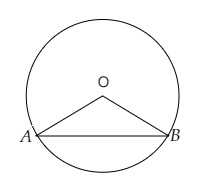# In a circle of radius $6 \mathrm{~cm}$, a chord of length $10 \mathrm{~cm}$ makes an angle of $110^{\circ}$ at the centre of the circle. Find the area of the sector $O A B$.

Given:

Radius of the circle $r=6 \mathrm{~cm}$.

Length of the arc $l=10 \mathrm{~cm}$.

Angle subtended at the centre $=110^{\circ}$.

To do:

We have to find the area of the sector $O A B$.

Solution:Let $OA$ and $OB$ are the radii of the circle and $AB$ the chord.

We know that,

Area of a sector is $\pi r^{2} (\frac{\theta}{360^{\circ}})$.

Therefore,

Area of the sector $OAB =\pi r^{2} \times \frac{\theta}{360^{\circ}}$

$= 3.14 \times 6 \times 6 \times \frac{110^{\circ}}{360^{\circ}} \mathrm{cm}^{2}$

$=36 \times 3.14 \times \frac{11}{36} \mathrm{~cm}^{2}$

$=34.54 \mathrm{~cm}^{2}$

The area of the sector $O A B$ is $34.54\ cm^2$.

Updated on: 10-Oct-2022

28 Views